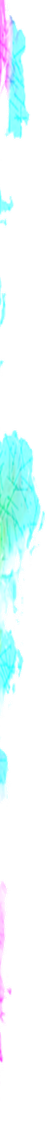### RegExp Quantifiers

• turned_in_notQuantifiers
• x{N}
• x{N,}
• x{N,M}
• x*
• x+
• x?

N : 0 or any positive number
M : any positive number

• To run below examples, try it inside `<script>` tag, in a basic html file

##### x{N}

It matches exactly N sequence of x's

For example :

``````var str="good food today";
var pattern=/o{2}/g;
// Output: oo,oo``````

Matches: good food today

##### x{N,}

It matches at least N sequence of x's

For example :

``````var str="good food today";
var pattern=/o{1,}/g;
// Output: oo,oo,o``````

Matches: good food today

##### x{N,M}

It matches N to M sequence of x's and N < M

For example :

``````var str="goood food today";
var pattern=/o{1,2}/g;
// Output: oo,o,oo,o``````

Matches: goood food today

##### x*

It matches zero or more occurrences of x
same as x{0,}

For example :

``````var str="books are goood friend";
var pattern=/o*d/g;
// Output: oood,d``````

Matches: books are goood friend

##### x+

It matches at least one x
same as x{1,}

For example :

``````var str="goood food today and ?";
var pattern=/o+d/g;
// Output: oood,ood,od``````

Matches: goood food today and ?

##### x?

It matches zero or one occurrences of x
same as x{0,1}

For example :

``````var str="goood food today and ?";
var pattern=/o?d/g;
// Output: od,od,od,d``````

Matches: goood food today and ?

##### Extra info

Works only when they are the end statements

x{N,}? = x{N}
x{N,M}? = x{N}
x{N}? = x{N}
x*? = x{0}
x+? = x{1}
x?? = x{0}

For example :

``````var str="dooo dooo do doo";
var pattern=/do{2,}?/g;